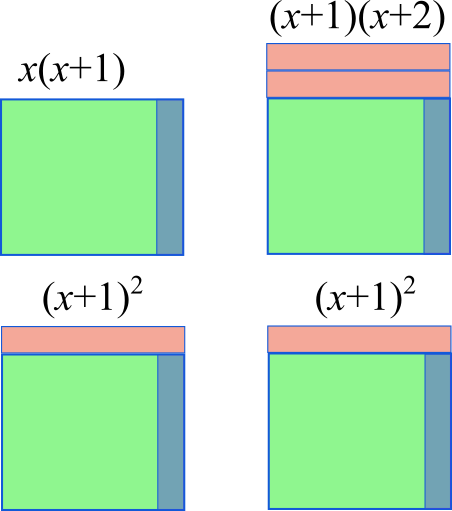#### You may also like### Summing Consecutive Numbers

15 = 7 + 8 and 10 = 1 + 2 + 3 + 4. Can you say which numbers can be expressed as the sum of two or more consecutive integers?### Always the Same

Arrange the numbers 1 to 16 into a 4 by 4 array. Choose a number. Cross out the numbers on the same row and column. Repeat this process. Add up you four numbers. Why do they always add up to 34?### Fibs

The well known Fibonacci sequence is 1 ,1, 2, 3, 5, 8, 13, 21.... How many Fibonacci sequences can you find containing the number 196 as one of the terms?

##### Age 11 to 14Challenge Level

1.
Amrit from Hymers College in the UK, Radi and Camilla and Viktor and Matija from European School Varese in Italy, Rubayat, Hondfa, Jacob and Nathan from Greenacre Public School in Australia, Ryan from Dulwich College Seoul in Korea and Peter from Durham Johnson School in the UK sent in good work on this problem.

Viktor and Matija spotted a numerical pattern and summarised it in words:

$2 \times 3 + 3 =9$
$5 \times 6 + 6 =36$
$4 \times 5 + 5 =25$
$9 \times 10 + 10 =100$
If you multiply the two consecutive numbers, which differ by one, then add the bigger number to that you're always going to get the square of the bigger number.

Ryan showed numerically why this works:
\begin{align}2 Ã— 3 + 3 = 9 &>>> 3 + 3 + 3 = 3 Ã— 3 = 9\\ 5 Ã— 6 + 6 = 36 &>>> 6 + 6 + 6 + 6 + 6 + 6 = 6 Ã— 6 = 36\\ 4 Ã— 5 + 5 = 25 &>>> 5 + 5 + 5 + 5 + 5 = 5 Ã— 5 = 25\\ 9 Ã— 10 + 10 = 100 &>>> 10 + 10 + 10 + 10 + 10 + 10 + 10 + 10 + 10+ 10 = 10 Ã— 10 = 100\end{align}

This is Amrit's algebraic proof:
Represented algrbraically, this is $n(n+1)+(n+1)$

Factorising, $(n+1)(n+1)$

And we see this is $(n+1)^2$

This is Radi and Camilla's diagram: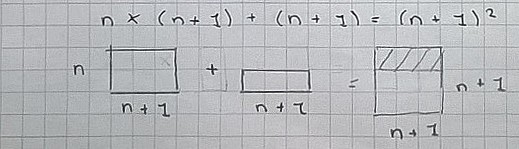2.
Amrit from Hymers College in the UK, Radi and Camilla and Viktor and Matija from European School Varese in Italy, Rubayat, Hondfa, Jacob and Nathan from Greenacre Public School in Australia and Peter from Durham Johnson School in the UK sent in good work on this problem.

This is Radi and Camilla's work: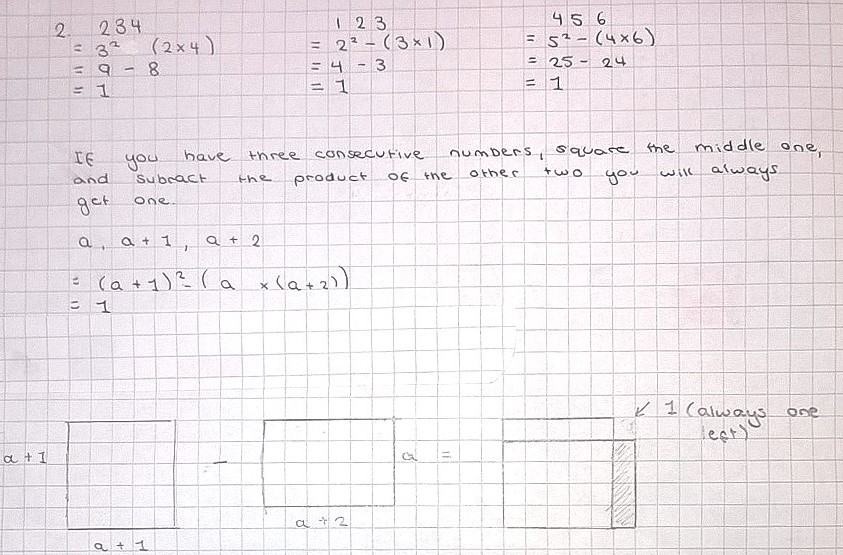3.
Amrit from Hymers College in the UK, Viktor and Matija and Tiago and Finn from European School Varese in Italy, Rubayat, Hondfa, Jacob and Nathan from Greenacre Public School in Australia, Marc and Yang from King's College Alicante in Spain and Peter from Durham Johnson School in the UK sent in good work on this problem.

Viktor and Matija spotted the numerical pattern:

$3\times3-1\times1=8$
$8\times8-6\times6=28$
$7\times7-5\times5=24$
$10\times10-8\times8=36$

Amrit put it in words:
If you take two numbers with a difference of 2 and subtract the square of the smaller one from the square of the bigger one, the result is four times the mean of the two numbers.

Tiago and Finn proved it algebraically:
\begin{align}(nÃ—n)-(n-2)Ã—(n-2)=&n^2-(n-2)Ã—(n-2)\\ =&n^2-(n^2-4n+4)\\ =&n^2-n^2+4n-4\\ =&4(n-1)\end{align}

The answer is always the sum of the digits [multiplied by 2]. $a\times a-b\times b=2b+2a$ if $a-b=2$

Marc and Yang called the numbers $x$ and $y$ and noticed that
$x^2 - y^2 = (x+y)(x-y)$

Amrit's proof used Marc and Yang's idea to prove Peter's representation in words.
$(x+2)^2-x^2=(x+2+x)(x+2-x)$, which simplifies to $(2x+2)(2)$

Tiago and Finn's diagram representation looked like this: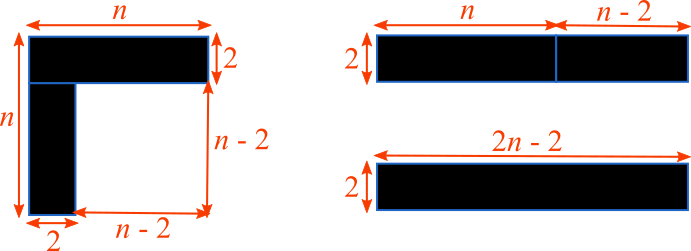4.
Peter from Durham Johnson School in the UK noticed that the expressions all simplify to 2:
$n(n+1)âˆ’(nâˆ’1)(n+2)=2$
$(n+1)(n+2)âˆ’n(n+3)=2$
$(nâˆ’3)(nâˆ’2)âˆ’(nâˆ’4)(nâˆ’1)=2$

The following observations can be made:
"If you choose four consecutive numbers and subtract from the product of the two middle ones the product of the other two, then you always get $2$."

General expression: $n(n+1) - (n-1)(n+2) = (n^2 + n) - (n^2 + n - 2) = 2$.

It follows that $(n+1)(n+2) - n(n+3)$ and  $(n-3)(n-2)-(n-4)(n-1)$ are always $2$.

These diagrams represent the process. The shaded area in the right hand diagram needs to be 'subtracted' from the green area.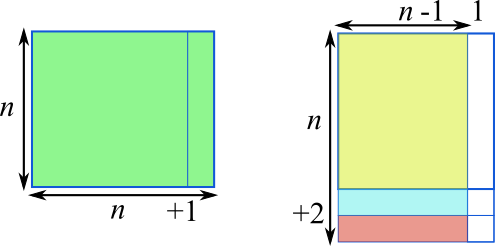This leaves a gap of 2 squares: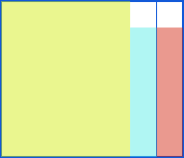5.
Maddy and Grace from the Stephen Perse Foundation in the UK continued the numerical pattern:
$2 \times 4 + 1 = 9$
$4 \times 6 + 1 = 25$
$5 \times 7 + 1 = 36$
$9 \times11 + 1 = 100$
$10 \times 12 + 1 = 121$
$20 \times 22 + 1 = 441$

Rubayat, Hondfa, Jacob and Nathan from Greenacre Public School in Australia noticed that
The pattern is what Charlie quoted,"If you multiply two numbers that differ by 2, and then add one, the answer is always the square of the number between them!" $3 \times 5 + 1= 16$ or $4^2$. $5 \times 7 + 1 =36$ or $6^2$.

Peter's proof was different to Charlie's:
$(n-1)(n+1)= n^2-1$, so $(n-1)(n+1)+1=n^2$

Chloe, Sophia and Shreya from North London Collegiate School made a clear diagram with a good explanation: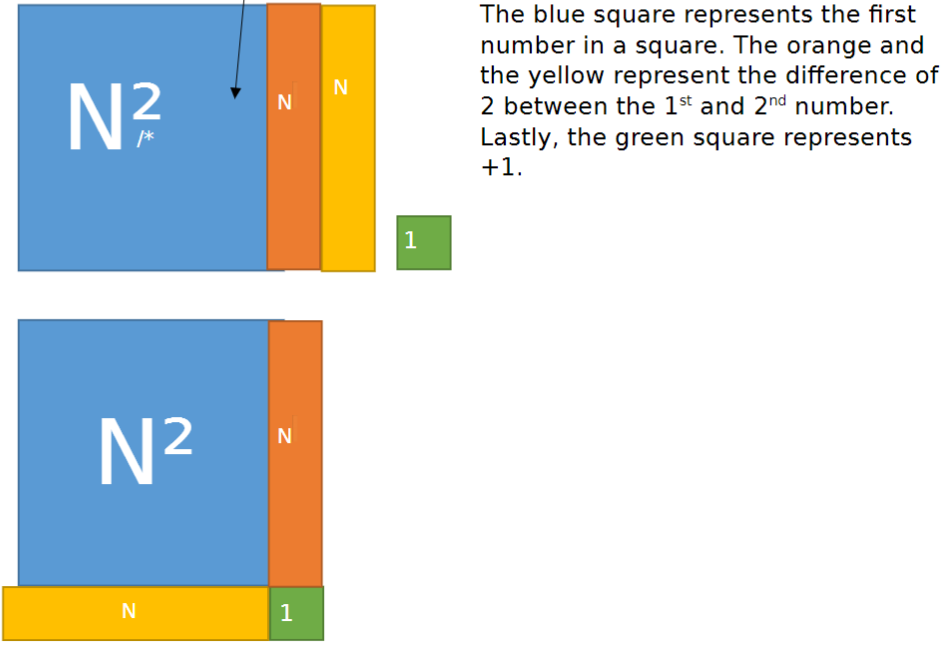6.
Rubayat, Hondfa, Jacob and Nathan represented the problem numerically and in words:
The result equals the middle number squared, then times by $2$. For example: $1,2,3.$ $1 \times 2=2,$ $2 \times 3=6$ therefore $6 +2 = 8$ [which is $2^2$ times $2$].
Another example is $50,51$ and $52.$ $50 \times 51= 2550,$ $51 \times 52= 2652.$ Therefore
$2550 + 2652=5202.$ $51$ squared then times $2$ equals $5202.$

Amrit proved it algebraically:
Represented algebraically, this is
$$x(x+1)+(x+1)(x+2)$$Factorising,$$(x+1)(x+x+2)$$Simplifying,$$(x+1)(2x+2)$$Factorising,$$2(x+1)(x+1)$$Simplifying,$$2(x+1)^2$$